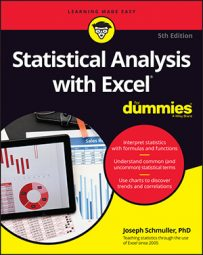##### Statistical Analysis with Excel For DummiesExcel can help you make all sorts of calculations. Here's a selection of Excel's statistical worksheet functions. Each one returns a value into a selected cell.

Check out these functions for central tendency and variability.

 Function What it calculates AVERAGE Mean of a set of numbers AVERAGEIF Mean of a set of numbers that meet a condition AVERAGEIFS Mean of a set of numbers that meet one or more conditions HARMEAN Harmonic mean of a set of positive numbers GEOMEAN Geometric mean of a set of positive numbers MODE.SNGL Mode of a set of numbers MEDIAN Median of a set of numbers VAR.P Variance of a set of numbers considered to be a population VAR.S Variance of a set of numbers considered to be a sample STDEV.P Standard deviation of a set of numbers considered to be a population STDEV.S Standard deviation of a set of numbers considered to be a sample STANDARDIZE A standard score based on a given mean and standard deviation
These handy functions for relative standing can also be very useful.
 Function What it calculates RANK.EQ Rank of a number in a set of numbers. If more than one number has the same rank, it returns the top rank of those numbers. RANK.AVG Rank of a number in a set of numbers. If more than one number has the same rank, it returns their average. PERCENTRANK.INC Rank of a number in a set of numbers, expressed as a percent of the numbers it's greater than or equal to. PERCENTRANT.EXC Rank of a number in a set of numbers, expressed as a percent of the numbers it's greater than. PERCENTILE.INC The indicated percentile in a set of numbers, in terms of "greater than or equal to." PERCENTILE.EXC The indicated percentile in a set of numbers, in terms of "greater than." QUARTILE.INC The 1st, 2nd, 3rd, or 4th quartile of a set of numbers, in terms of "greater than or equal to." QUARTILE.EXC The 1st, 2nd, 3rd, or 4th quartile of a set of numbers, in terms of "greater than."
These functions for correlation and regression are also good ones to know.
 Function What it Calculates CORREL Correlation coefficient between two sets of numbers PEARSON Same as CORREL. (Go figure!) RSQ Coefficient of determination between two sets of numbers (square of the correlation coefficient) SLOPE Slope of a regression line through two sets of numbers INTERCEPT Intercept of a regression line through two sets of numbers STEYX Standard error of estimate for a regression line through two sets of numbers